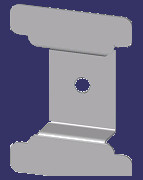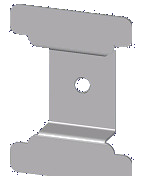Welcome to bytebang » The blog about all and nothing » Removing a uniform background from an image with Java

# The Problem

QAF files are often used in PLM Systems (like Siemens Teamcenter) to give a preview of the stored CAD dataset. Under the hood they are plain TIFF or BMP images which are just renamed. This means that you can edit them with any common image manipulation software like GIMP.

Unfortunately some Teamcenter CAD integrations are producing quiet 'ugly' previews - like this one:If you want to have (for obvious reasons) a transparent background instead of the violet one, and it is not possible to change the default background color in the Teamcenter integration then you have to remove the background progamatically.

# The Solution

The solution seems to be simple:

1. Find the background pixels
2. Make them transparent.

The first question which has to be answered: What is the color of the background ?
Well, In my test images the background was always violet. So the trivial approach was to find out the RGV values with a image manipulation software, but I decided to choose the top left pixel as reference.

Color leftTopColor = new Color(this.img.getRGB(0, 0));

It would be be better to choose more than one pixel for background identification (something linke the mean RGB  of all pixels at the border), but in my case this was sufficient.

### Approach#1: Finding background pixels by RGB value

The given image has a (nearly) uniform violet background. So all we have to do, is to find all pixels which have the same color: Sounds great, donesn't work !

Source image Filtered ImageThis is the reason for the violet surrounding is, that due some compression artifacts within the qaf file the viloet pixels are not equally violet. So we need another approach: Lets find all pixels which have nearly the same colour as the top left pixel.The problem is, that in the RGB color space similar colors are hard to identify. I tried to do this via threshold values, but the results where not convincing.

### Approach#2: Finding background pixels by HSV and some threshold

To find similar colors I had to convert the RGB value of each pixel into the HSV colorspace:The H (=hue) represents the color. E.g. All green colors have (compared to yellow) a similar H component. The S (=Saturation) and V (=Value) re representing the other aspects of this colorspace.

To make it a little bit more configurable, i decided to write class which extends RGBImageFilter and can be used to identify similar colors:

/**
* Filter to make similar colours transparent
* @author bytebang
*/

private class SimilarColorFilter extends RGBImageFilter
{
Double delta_hue;
Double delta_saturation;
Double delta_brightness;

float thue;
float tsaturation;
float tbrightness;

public SimilarColorFilter(Color color, Double delta_hue, Double delta_saturation, Double delta_brightness)
{
this.delta_hue = delta_hue;
this.delta_saturation = delta_saturation;
this.delta_brightness = delta_brightness;

// Convert to other colourspace
int r,g,b;
r = color.getRed();
g = color.getGreen();
b = color.getBlue();

float[] hsv = new float;
Color.RGBtoHSB(r,g,b,hsv);

// Merken der aktuellen Farbparameter
this.thue = hsv;
this.tsaturation = hsv;
this.tbrightness = hsv;
}

@Override
public int filterRGB(int x, int y, int rgb)
{
Color cpix = new Color(rgb);
float[] hsv = new float;
Color.RGBtoHSB(cpix.getRed(),cpix.getGreen(),cpix.getBlue(), hsv);

float phue = hsv;
float psaturation = hsv;
float pbrightness = hsv;

if (isInTolerance(phue, this.thue, this.delta_hue) &&
isInTolerance(psaturation, this.tsaturation, this.delta_saturation) &&
isInTolerance(pbrightness, this.tbrightness, this.delta_brightness))
{
// Mark the alpha bits as zero - transparent
return 0x00FFFFFF & rgb;
}
else
{
// nothing to do
return rgb;
}
}

/**
* Returns true if the value is smaller than delta.
* If delta == null, then it returns true
*
* @param actual_value actual value
* @param target_value desired value
* @param max_delta max difference or null
* @return true if is in tolerance
*/

public boolean isInTolerance(double actual_value, double target_value, Double max_delta)
{
if(max_delta == null)
{
return true;
}

double low = Math.min(actual_value, target_value);
double high = Math.max(actual_value, target_value);

return (high-low) <= max_delta;
}
}

If you apply this filter with the correct thresholds, then re results are acceptable:

ImageFilter filter = new SimilarColorFilter(leftTopColor, 0.5, 0.2, 0.06);

This means that the maximum differences in hue can be up to 50% from the background color, in saturation 20% and in value 6%.

Source image Filtered ImageIt seems to work pretty well. The complete code can be found on GitHub in my repository: https://github.com/bytebang/qaf-transparency

Happy coding.

# Get Social

 Tweet

(c) 2023, by bytebang e.U. - Impressum - Datenschutz / Nutzungsbedingungen
-